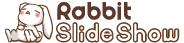# Self-testing Code in Ruby

2017-10-16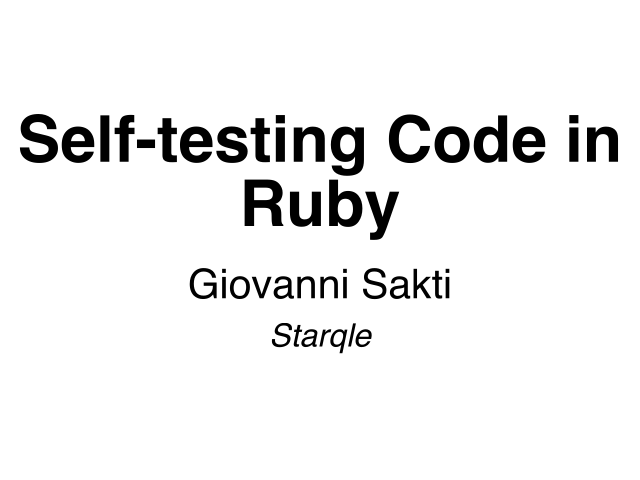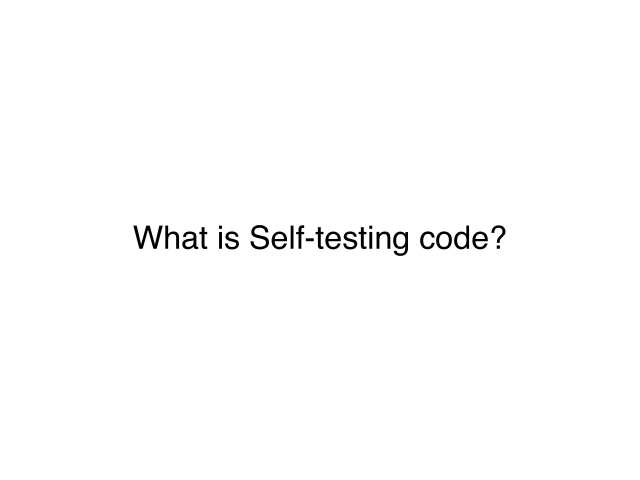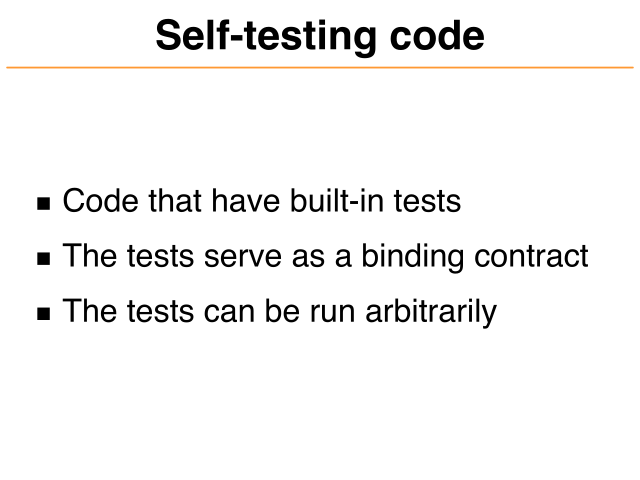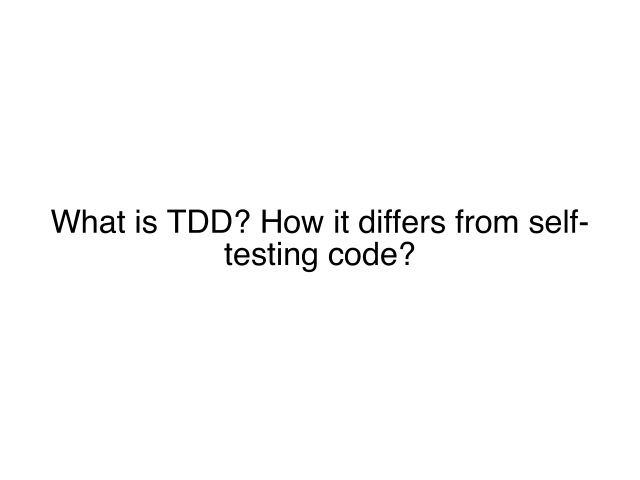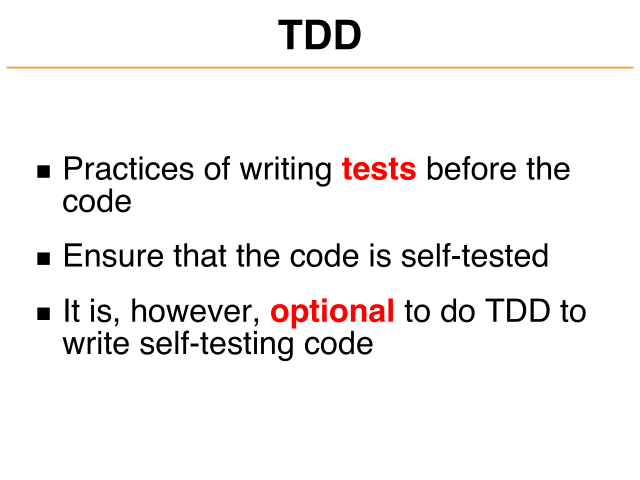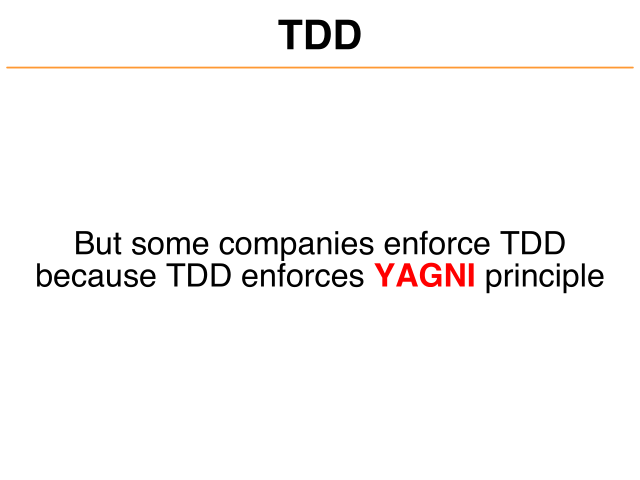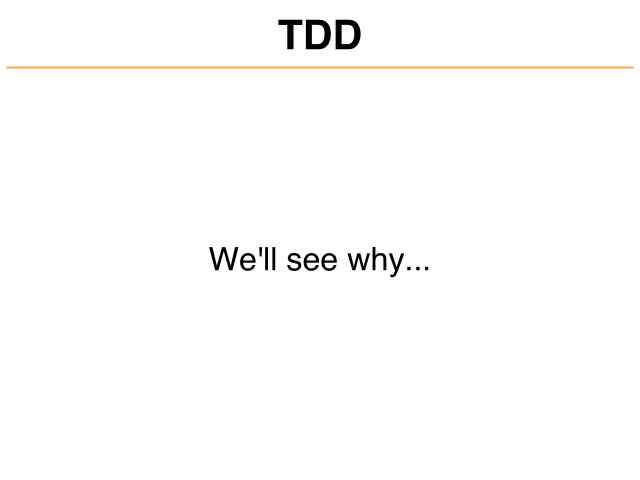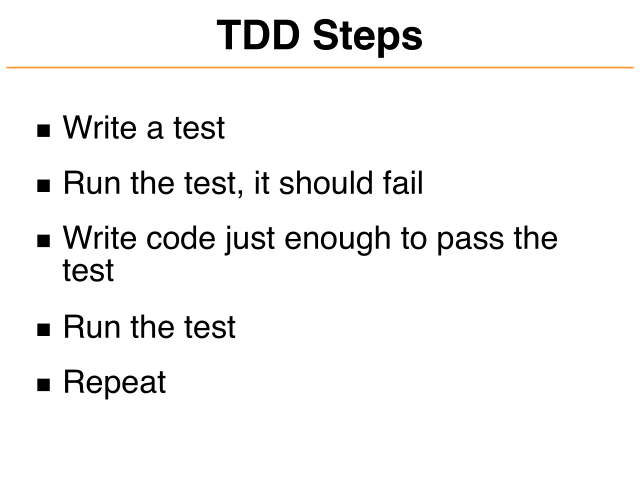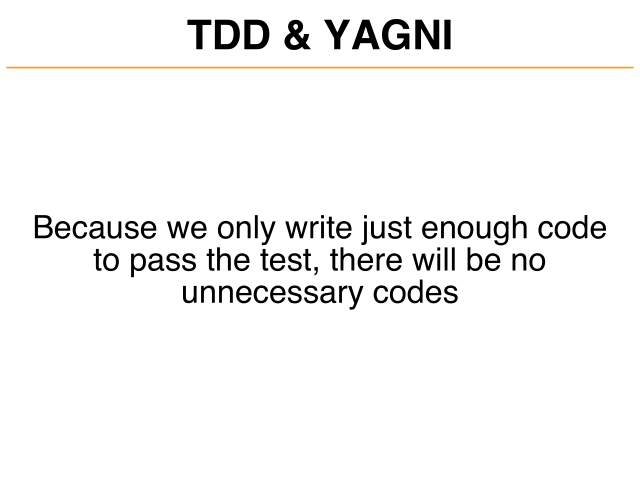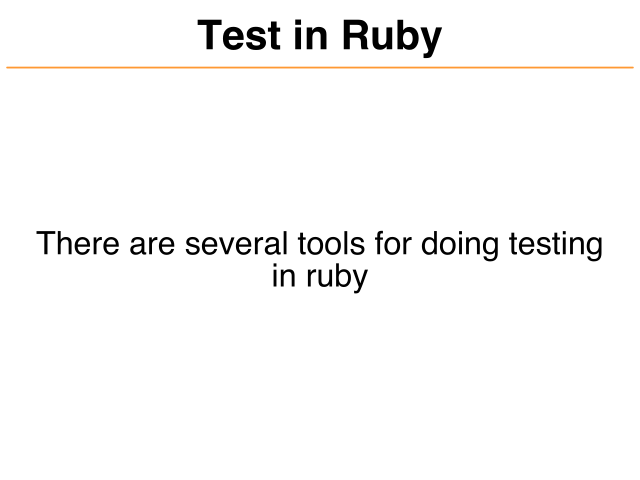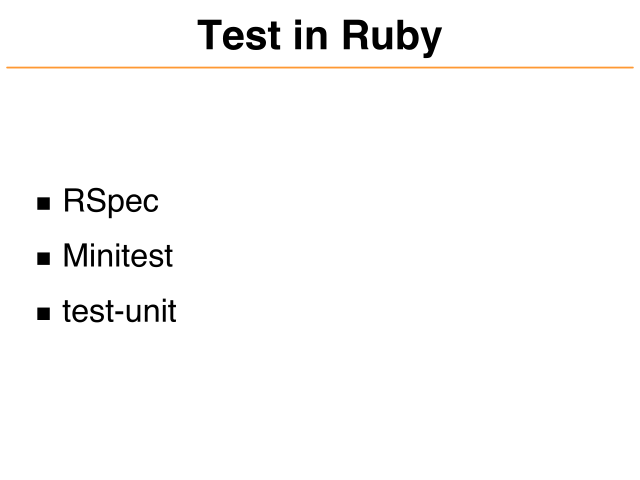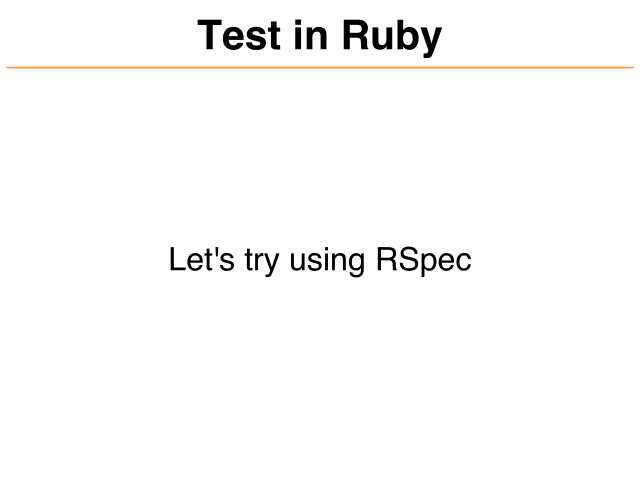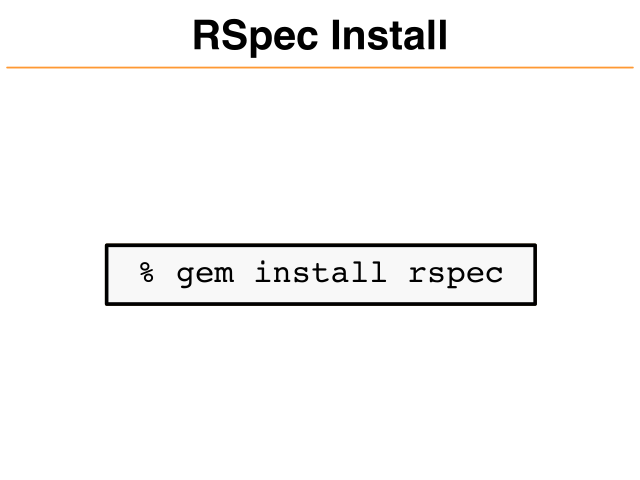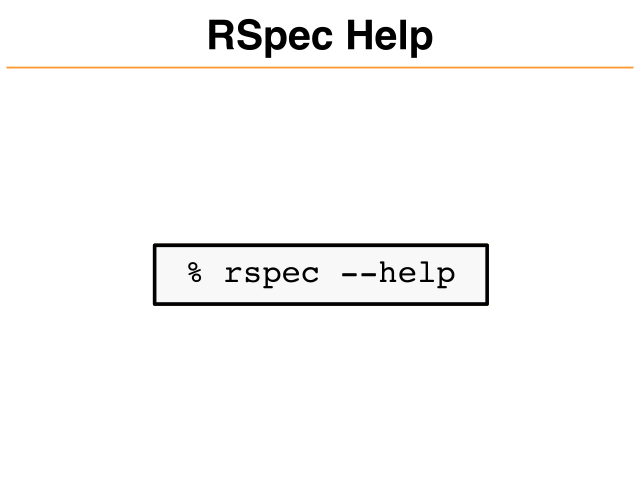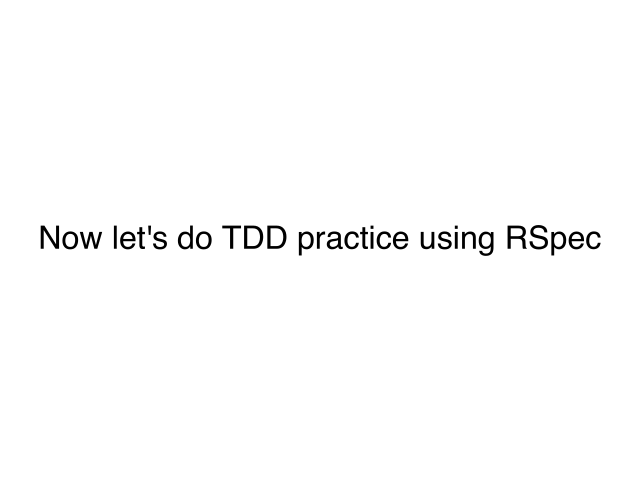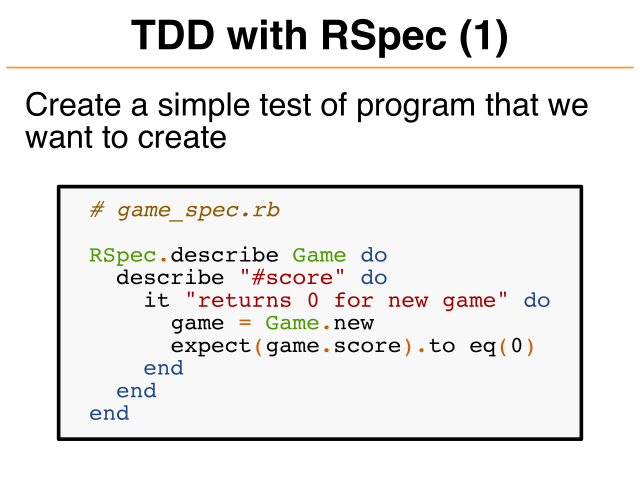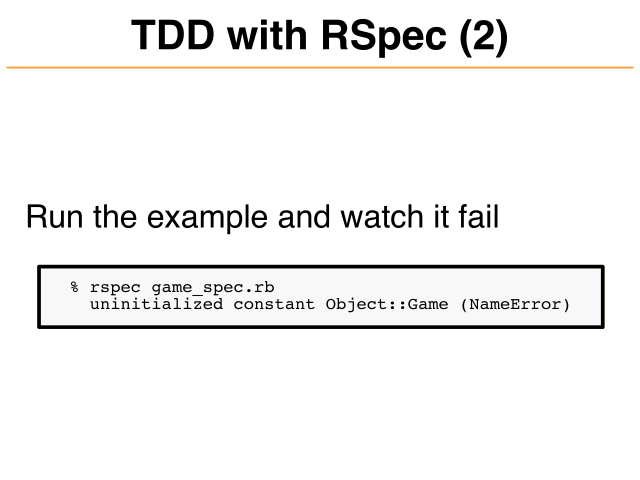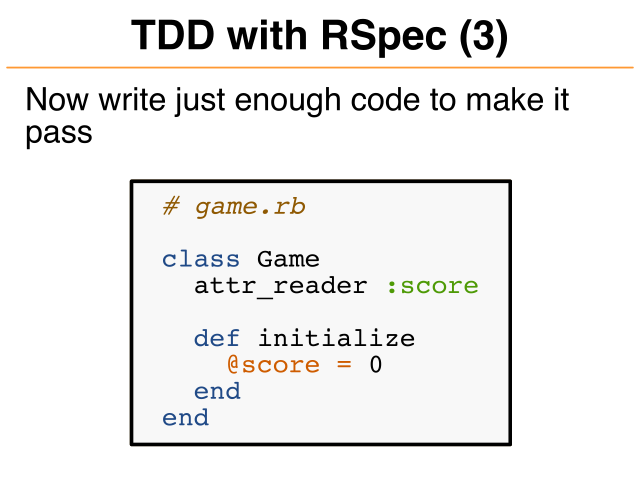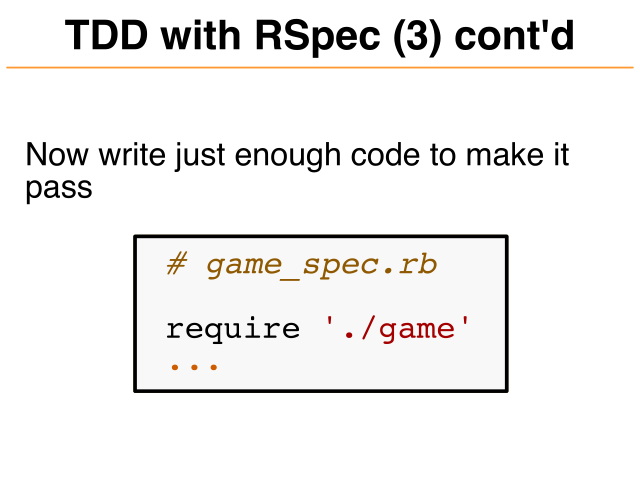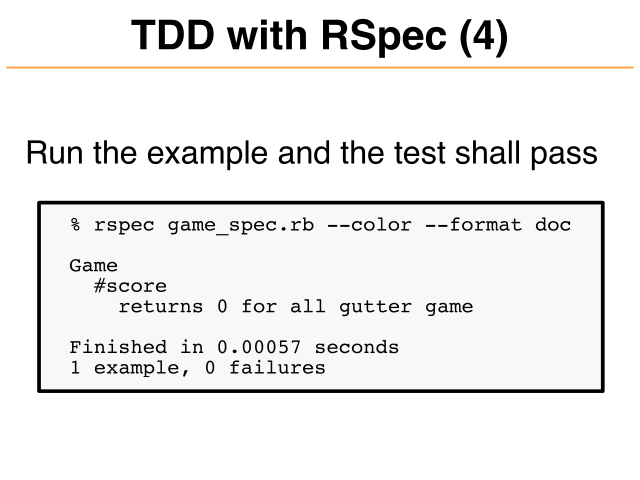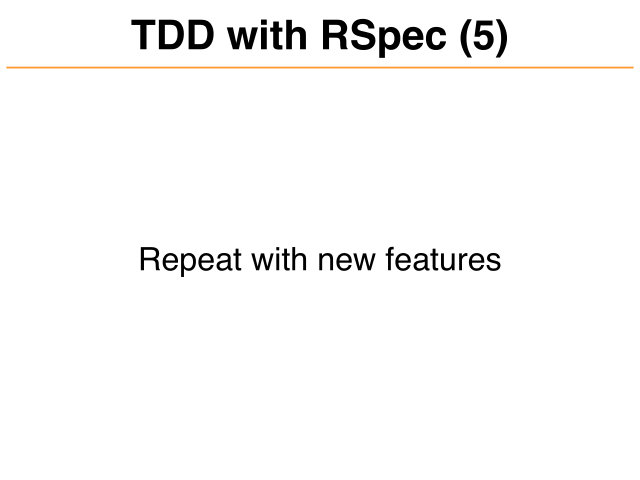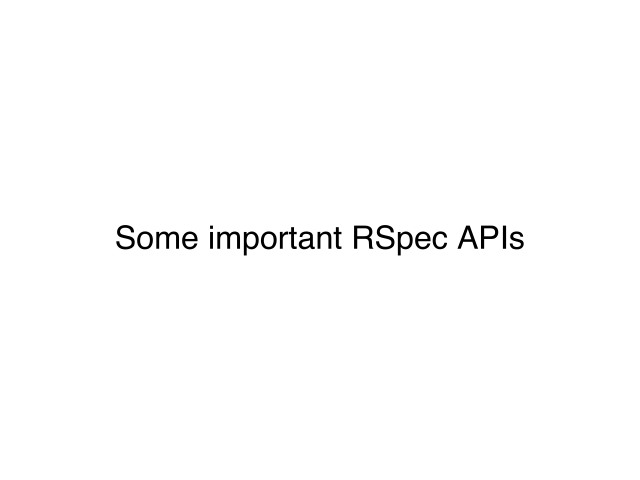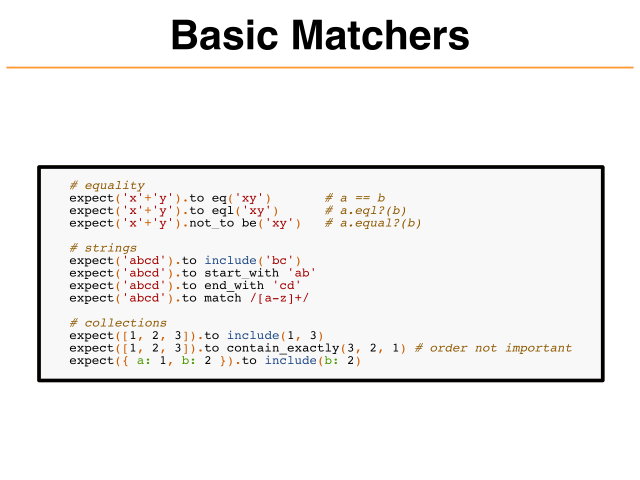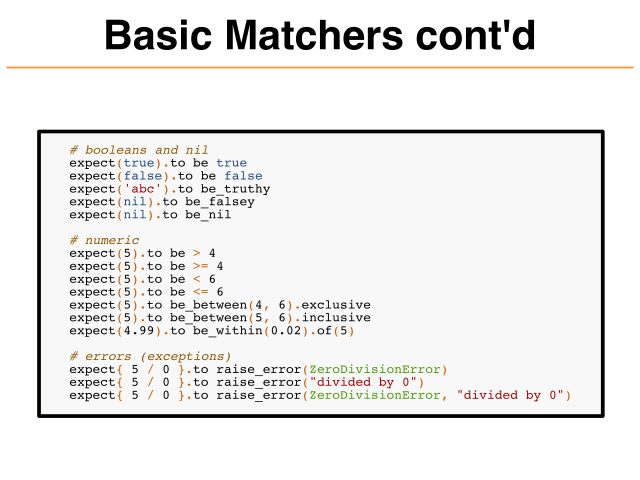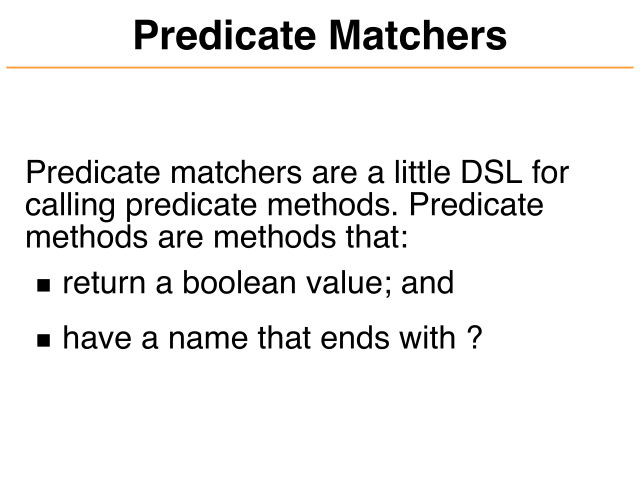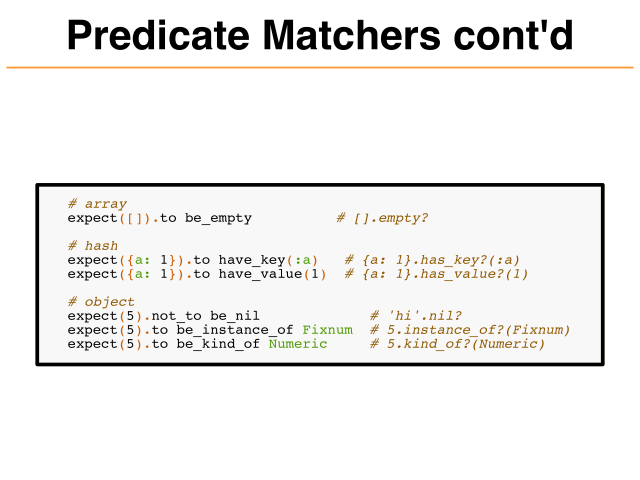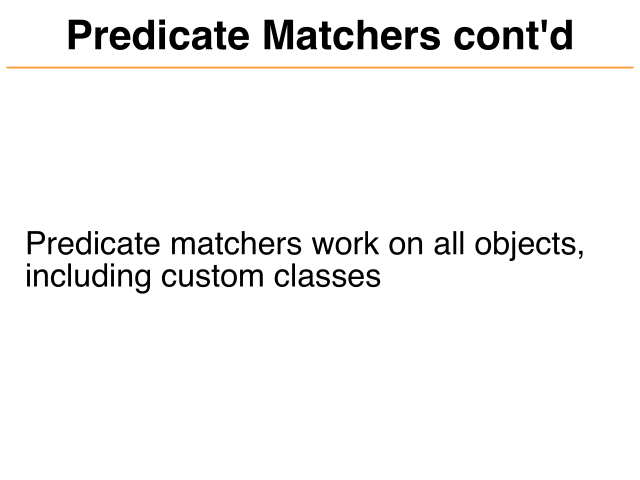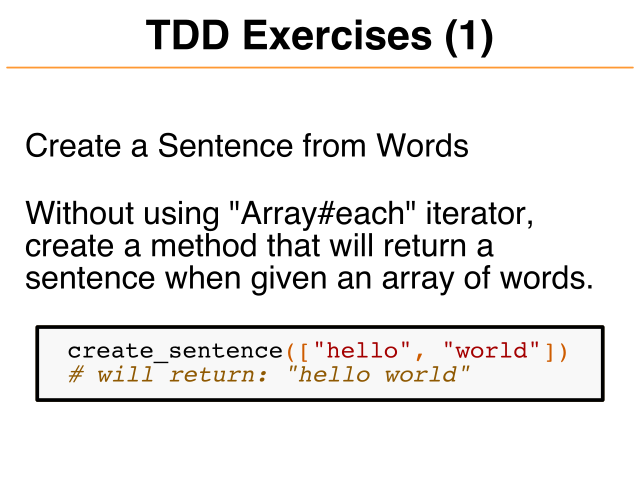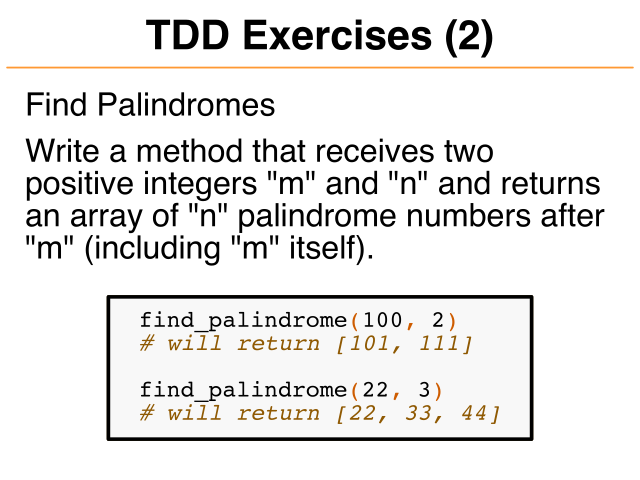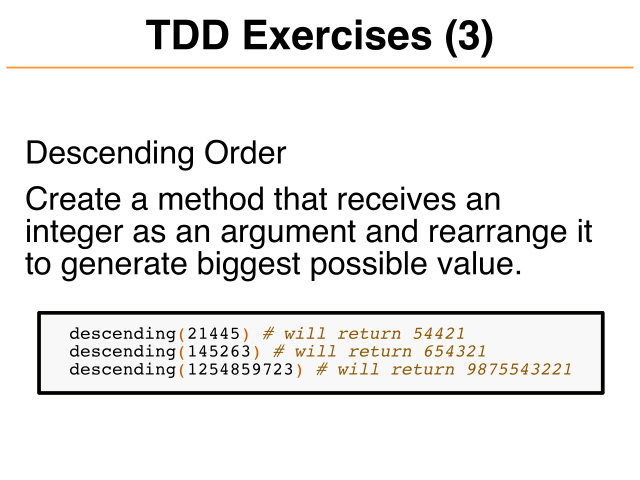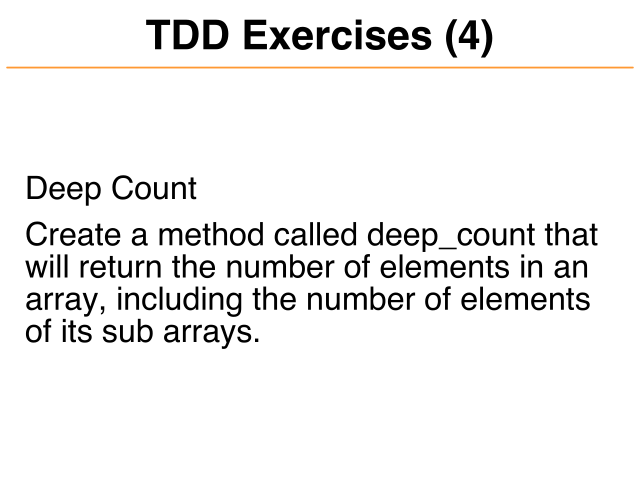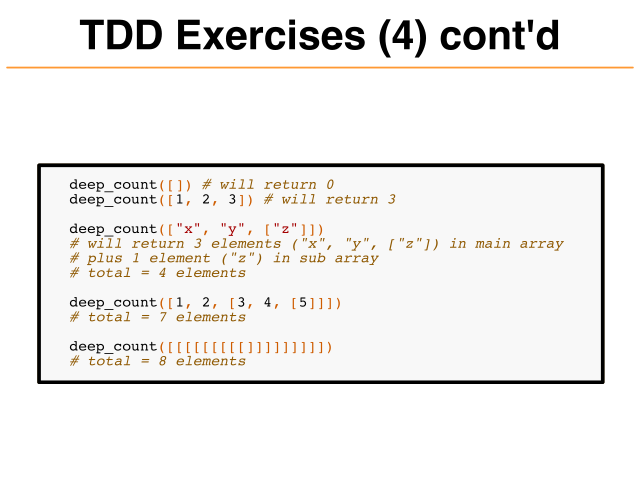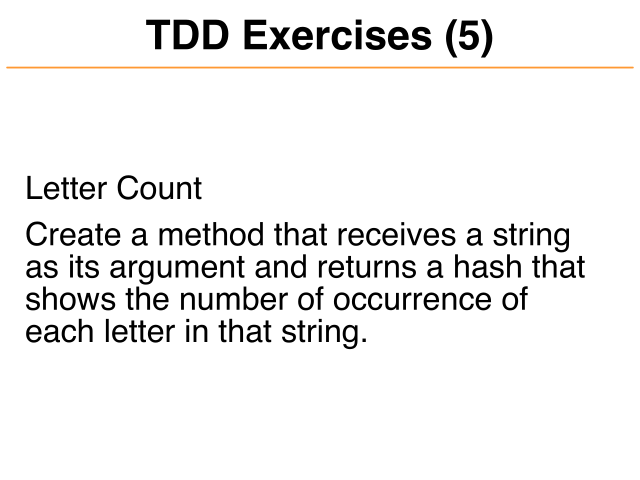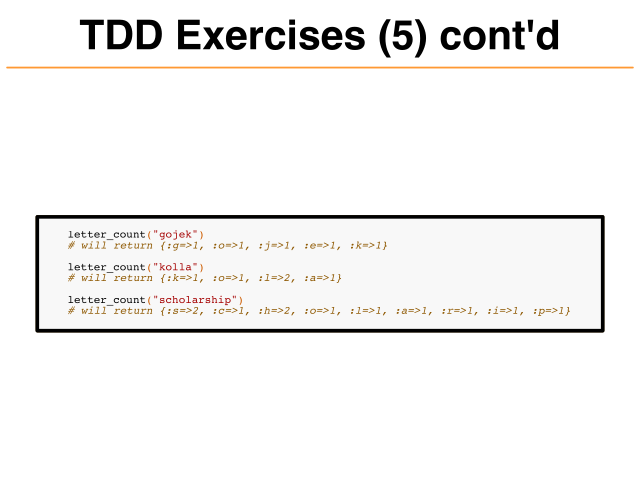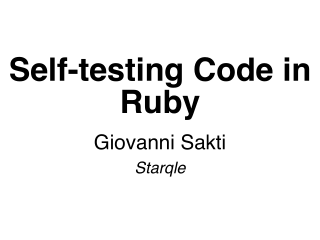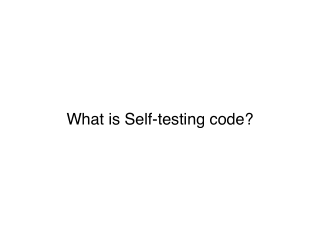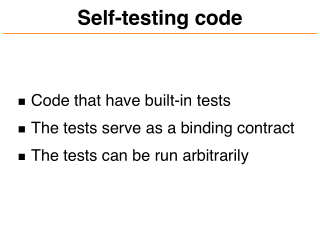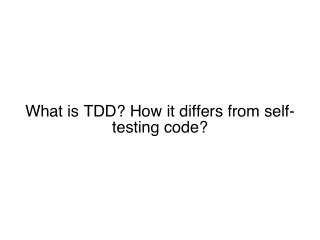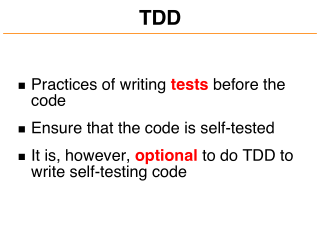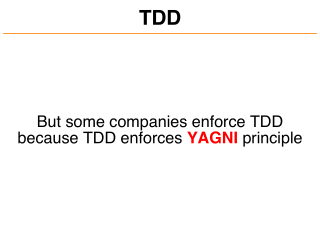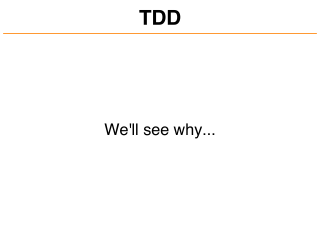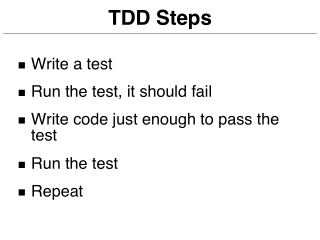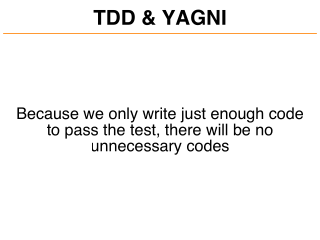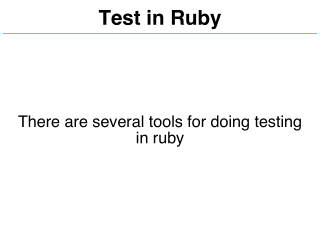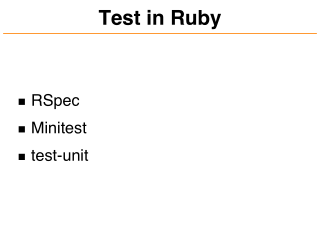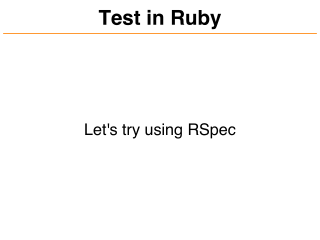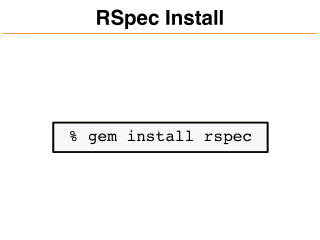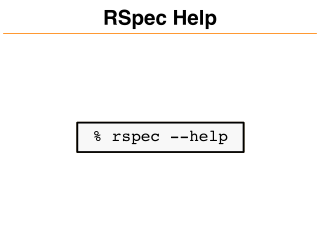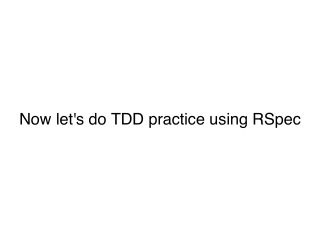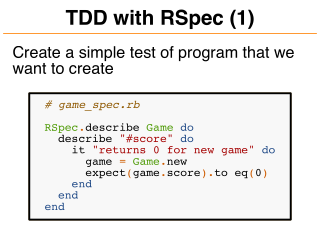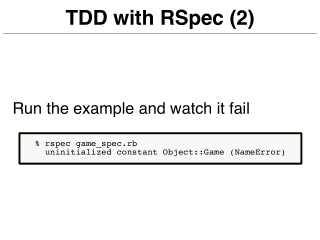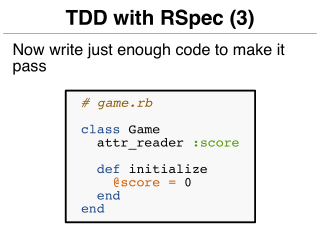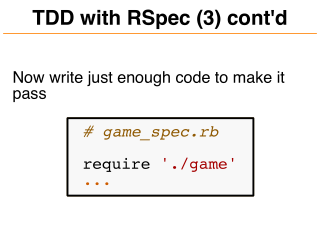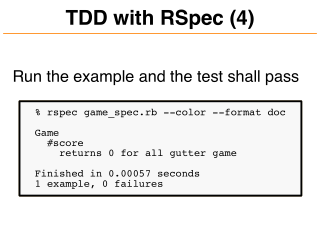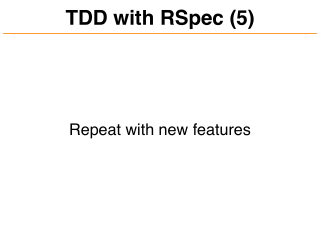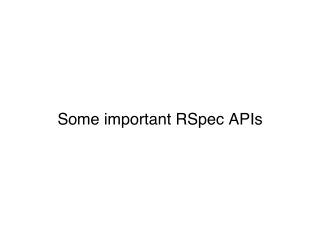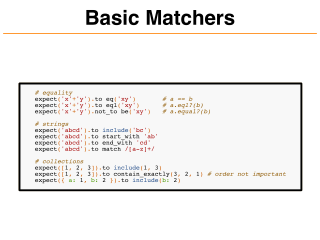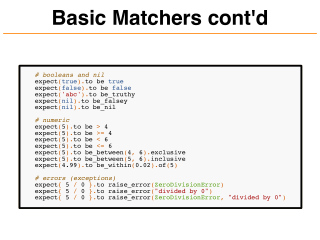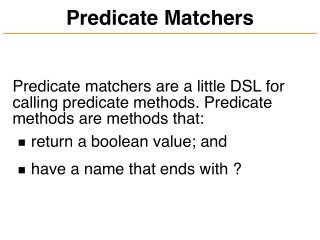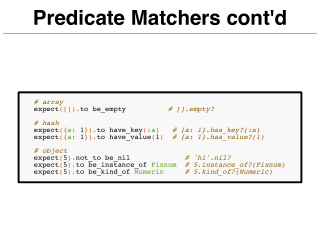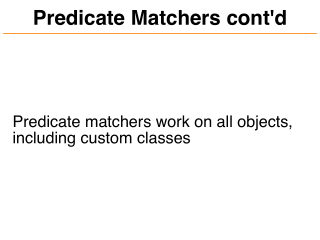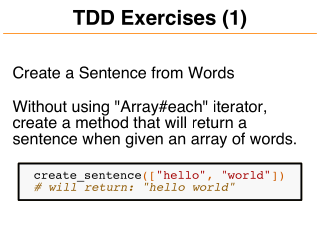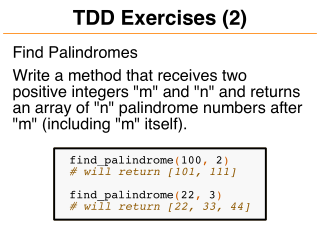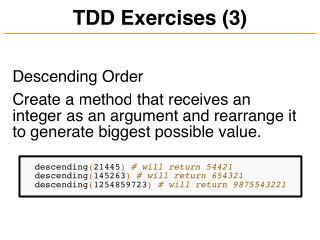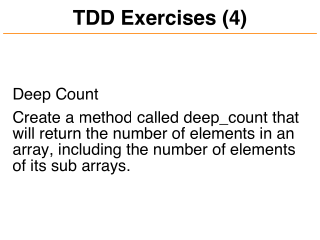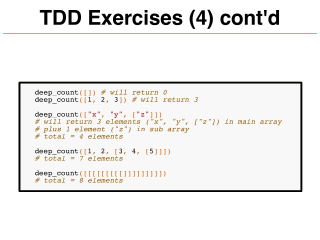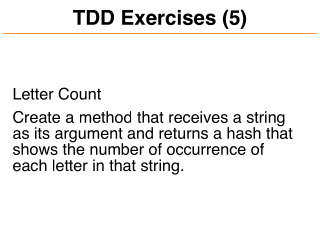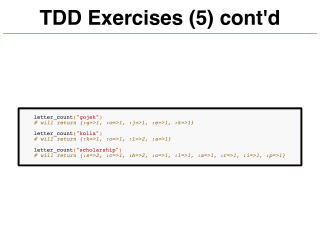## Description

This talk explains the concept of self-testing code with practices in ruby using rspec.

## Text

### Page: 1

```Self-testing Code in
Ruby
Giovanni Sakti
Starqle```

### Page: 2

`What is Self-testing code?`

### Page: 3

```Self-testing code
Code that have built-in tests
The tests serve as a binding contract
The tests can be run arbitrarily```

### Page: 4

```What is TDD? How it differs from self-
testing code?```

### Page: 5

```TDD
Practices of writing tests before the
code
Ensure that the code is self-tested
It is, however, optional to do TDD to
write self-testing code```

### Page: 6

```TDD
But some companies enforce TDD
because TDD enforces YAGNI principle```

### Page: 7

```TDD
We'll see why...```

### Page: 8

```TDD Steps
Write a test
Run the test, it should fail
Write code just enough to pass the
test
Run the test
Repeat```

### Page: 9

```TDD & YAGNI
Because we only write just enough code
to pass the test, there will be no
unnecessary codes```

### Page: 10

```Test in Ruby
There are several tools for doing testing
in ruby```

### Page: 11

```Test in Ruby
RSpec
Minitest
test-unit```

### Page: 12

```Test in Ruby
Let's try using RSpec```

### Page: 13

```RSpec Install
% gem install rspec```

### Page: 14

```RSpec Help
% rspec --help```

### Page: 15

`Now let's do TDD practice using RSpec`

### Page: 16

```TDD with RSpec (1)
Create a simple test of program that we
want to create
# game_spec.rb
RSpec.describe Game do
describe "#score" do
it "returns 0 for new game" do
game = Game.new
expect(game.score).to eq(0)
end
end
end```

### Page: 17

```TDD with RSpec (2)
Run the example and watch it fail
% rspec game_spec.rb
uninitialized constant Object::Game (NameError)```

### Page: 18

```TDD with RSpec (3)
Now write just enough code to make it
pass
# game.rb
class Game
def initialize
@score = 0
end
end```

### Page: 19

```TDD with RSpec (3) cont'd
Now write just enough code to make it
pass
# game_spec.rb
require './game'
...```

### Page: 20

```TDD with RSpec (4)
Run the example and the test shall pass
% rspec game_spec.rb --color --format doc
Game
#score
returns 0 for all gutter game
Finished in 0.00057 seconds
1 example, 0 failures```

### Page: 21

```TDD with RSpec (5)
Repeat with new features```

### Page: 22

`Some important RSpec APIs`

### Page: 23

```Basic Matchers
# equality
expect('x'+'y').to eq('xy')
expect('x'+'y').to eql('xy')
expect('x'+'y').not_to be('xy')
# strings
expect('abcd').to
expect('abcd').to
expect('abcd').to
expect('abcd').to
# a == b
# a.eql?(b)
# a.equal?(b)
include('bc')
start_with 'ab'
end_with 'cd'
match /[a-z]+/
# collections
expect([1, 2, 3]).to include(1, 3)
expect([1, 2, 3]).to contain_exactly(3, 2, 1) # order not important
expect({ a: 1, b: 2 }).to include(b: 2)```

### Page: 24

```Basic Matchers cont'd
# booleans and nil
expect(true).to be true
expect(false).to be false
expect('abc').to be_truthy
expect(nil).to be_falsey
expect(nil).to be_nil
# numeric
expect(5).to be > 4
expect(5).to be >= 4
expect(5).to be < 6
expect(5).to be <= 6
expect(5).to be_between(4, 6).exclusive
expect(5).to be_between(5, 6).inclusive
expect(4.99).to be_within(0.02).of(5)
# errors (exceptions)
expect{ 5 / 0 }.to raise_error(ZeroDivisionError)
expect{ 5 / 0 }.to raise_error("divided by 0")
expect{ 5 / 0 }.to raise_error(ZeroDivisionError, "divided by 0")```

### Page: 25

```Predicate Matchers
Predicate matchers are a little DSL for
calling predicate methods. Predicate
methods are methods that:
return a boolean value; and
have a name that ends with ?```

### Page: 26

```Predicate Matchers cont'd
# array
expect([]).to be_empty
# hash
expect({a: 1}).to have_key(:a)
expect({a: 1}).to have_value(1)
# [].empty?
# {a: 1}.has_key?(:a)
# {a: 1}.has_value?(1)
# object
expect(5).not_to be_nil
expect(5).to be_instance_of Fixnum
expect(5).to be_kind_of Numeric
# 'hi'.nil?
# 5.instance_of?(Fixnum)
# 5.kind_of?(Numeric)```

### Page: 27

```Predicate Matchers cont'd
Predicate matchers work on all objects,
including custom classes```

### Page: 28

`Now let's do some exercises...`

### Page: 29

```TDD Exercises (1)
Create a Sentence from Words
Without using "Array#each" iterator,
create a method that will return a
sentence when given an array of words.
create_sentence(["hello", "world"])
# will return: "hello world"```

### Page: 30

```TDD Exercises (2)
Find Palindromes
Write a method that receives two
positive integers "m" and "n" and returns
an array of "n" palindrome numbers after
"m" (including "m" itself).
find_palindrome(100, 2)
# will return [101, 111]
find_palindrome(22, 3)
# will return [22, 33, 44]```

### Page: 31

```TDD Exercises (3)
Descending Order
Create a method that receives an
integer as an argument and rearrange it
to generate biggest possible value.
descending(21445) # will return 54421
descending(145263) # will return 654321
descending(1254859723) # will return 9875543221```

### Page: 32

```TDD Exercises (4)
Deep Count
Create a method called deep_count that
will return the number of elements in an
array, including the number of elements
of its sub arrays.```

### Page: 33

```TDD Exercises (4) cont'd
deep_count([]) # will return 0
deep_count([1, 2, 3]) # will return 3
deep_count(["x", "y", ["z"]])
# will return 3 elements ("x", "y", ["z"]) in main array
# plus 1 element ("z") in sub array
# total = 4 elements
deep_count([1, 2, [3, 4, ]])
# total = 7 elements
deep_count([[[[[[[[[]]]]]]]]])
# total = 8 elements```

### Page: 34

```TDD Exercises (5)
Letter Count
Create a method that receives a string
as its argument and returns a hash that
shows the number of occurrence of
each letter in that string.```

### Page: 35

```TDD Exercises (5) cont'd
letter_count("gojek")
# will return {:g=>1, :o=>1, :j=>1, :e=>1, :k=>1}
letter_count("kolla")
# will return {:k=>1, :o=>1, :l=>2, :a=>1}
letter_count("scholarship")
# will return {:s=>2, :c=>1, :h=>2, :o=>1, :l=>1, :a=>1, :r=>1, :i=>1, :p=>1}```

`Thanks`